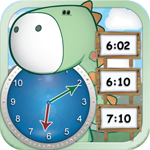## 5th Grade Printable Math Quiz SheetsThis page offers a number of math worksheets for Grade 5 based on Math Essentials math textbook and common core standards. Unit 1 covers the following topics: – fractions – simplify fractions – addition and subtraction of fractions – word problems with fractions Tap/click the following links to print math tests from Unit 1: 1. 5th Grade Unit 1 […]

## Tick Tock Clock – Learn to Tell Time UsiThe underlying teaching principle for Tick Tock Clock application is to help children connect the concepts of digital and mechanical clocks. It is assumed that a child knows how to read numbers which should make telling time on the digital clock easy. Comprehending the concept of the time passing is a process. Time is not […]